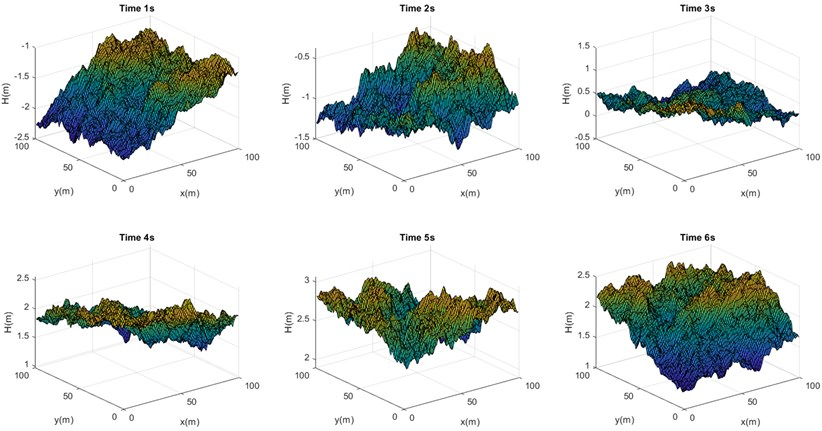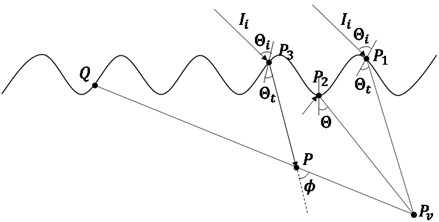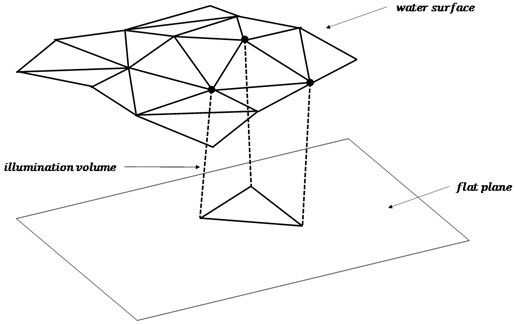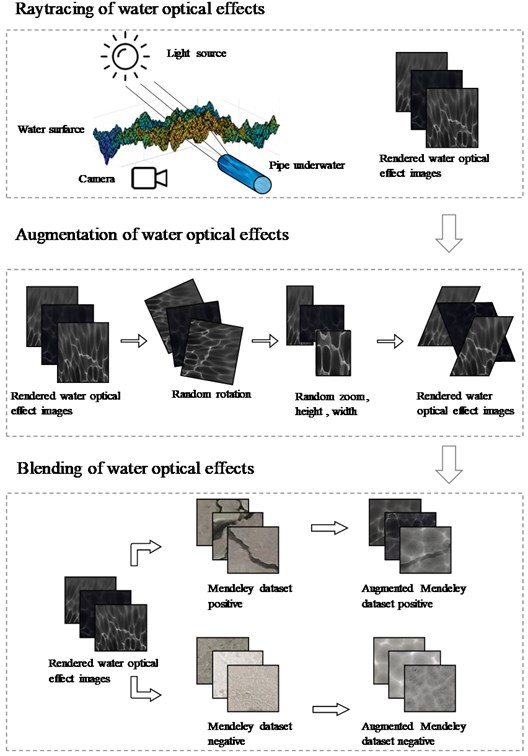Vibroengineering PROCEDIA

Published: 25 August 2022

# A deep learning-based approach for automatic detection of concrete cracks below the waterline

Ugnė Orinaitė1
Paulius Palevičius2
Mayur Pal3
Minvydas Ragulskis4
1, 2, 3, 4Department of Mathematical Modeling, Faculty of Mathematics and Natural Sciences, Kaunas University of Technology, Kaunas, Lithuania
Corresponding Author:
Ugnė Orinaitė
Views 49

#### Abstract

Convolutional neural networks have been created as deep learning-based approaches to automatically analyze photographs of concrete surfaces for crack diagnosis applications. Although deep learning-based systems assert to have extremely high accuracy, they frequently overlook how difficult it is to acquire images. Complex lighting situations, shadows, the irrationality of crack forms and widths, imperfections, and concrete spall frequently have an influence on real-world photos. The focus of the published research and accessible shadow databases is on photographs shot in controlled laboratory settings. In this research, we investigate the challenging underwater optical effects settings and the complexity of image classification for concrete crack detection. This research elaborates on difficulties encountered when using deep learning-based techniques to identify concrete cracks when optical effects are present. To improve the precision of automatically detecting concrete cracks on underwater surfaces, new optical effect augmentation techniques have been developed.

## 1. Introduction

Starlight entering the Earth's atmosphere causes several occurrences. The environment causes the light to be twisted, diffused, muted, and reddened. It is notably helpful to assess refraction, extinction, color effects, and the polarizing characteristic of the atmosphere using the Sun as a research instrument for atmospheric phenomena as presented in . The power of the “flying shadows” produced by air turbulence on the ground varies since they are both transported by the wind and constantly changing. Additionally, an examination of the scintillation signals captured by telescopes with various diameters and obscurations is presented, as well as a description of the structure of these flying shadows (secondary mirrors). Techniques for reducing scintillation “noise” are necessary for applications demanding tough photometry (such star microvariability) . The qualities of the scintillation are defined statistically, and the correlations between these attributes and the zenith angle of the star, the bandwidth of the light, and other variables are investigated. Because turbulence in the Earth’s atmosphere causes the stars to shine, some characteristics of the turbulence's structure may be inferred from measurements .

As the observation of stars in the sky, the study of space objects is hindered by atmospheric pressure, similarly, difficulties arise in the study of underwater objects due to the appearance of light refraction, reflection, caustics and other optical effects. It is extremely important to be able to detect concrete cracks regardless of the optical water illumination effects. Early identification of a potential collapse of underwater concrete structures, bridges, concrete pillars, and concrete pipelines enables the implementation of preventative measures to forestall failures, which can save property as well as lives. This work is intended to demonstrate that it is possible to use machine vision algorithms for robust underwater crack detection. In order to achieve this goal underwater optical effects are artificially generated and superimposed on the concrete images, which are then used for development of deep learning methodology for image classification using AlexNet .

The paper is organized as follows: Section 2 describes the method and material used in development of the underwater optical effects on concrete surfaces. Image augmentation technique is described in detail in this section. Section 3 presents the summary of deep learning model used for training and testing. Results are presented in Section 4. Finally, conclusions follow in Section 5.

## 2. Methods and materials

### 2.1. Mathematical models for the sea surface

Different propagation events are influenced by the light depending on the topography of the seabed and streamer depth. The scenarios are used to anticipate how the optical effects might impact the underwater pictures by feeding them into a model for underwater wave propagation.

Wave models are increasingly employed in a variety of coastal areas to research and predict wave conditions. They can be structured using both wave action models (also known as phase averaging models) and momentum models (or phase resolving models). The third generation of phase averaging models is used in a wider range of applications. Many advanced professional engineering applications, such as flow simulations and wave modeling, are available through commercially available software. Not all prepared and accessible models, meanwhile, are adjusted to the photos used to identify underwater cracks.

The basis for modeling ocean waves is the linear (or small-amplitude) wave theory of Airy , which is commonly used in marine engineering and computer graphics (Peachey . It describes sinusoidal waves, which are connected to clear skies. Trochoid models made by von Gerstner  and by Fournier and Reeves  were used to make their description more accurate (Rankine) . Random waves are produced by combining numerous waves and applying an equation to a 2D surface. Another method is the 2D inverse discrete Fourier transform, which has been used by Premože and Ashikhmin , Mastin et al. , Tessendorf  and others.

Oceanographers are more concerned about wave energy than amplitude. They do this by using a different type of spectrum, $S\left(w\right)$, sometimes referred to as a wave spectrum or a frequency energy spectrum, which offers information on the distribution of wave energy as a continuous function of frequency . Taking wind speed into consideration, Pierson and Moskowitz  developed a formula for the sea. However, the JONSWAP spectrum has been shown to be more appropriate for a fetch-limited sea with rising waves (Hasselmann et al.) :

1

where ${\omega }_{p}$ is the frequency of the peak of the spectrum. Usual value for ${\omega }_{p}$ is equal to ${\omega }_{p}=0.855g/{U}_{10}$, where ${U}_{10}$ is the speed of wind at the height of 10 meters above the sea surface. Here $\gamma$ is the peak enhancement factor, parameter $\sigma$ depicts the width of the peak. The values for the parameters in this spectrum equation are:

where $F$ is the fetch in meters, usually $\gamma =$ 3.3, but can vary from 1 to 7, and:

Since the fetch is an essential part of the description of the sea state, we use the JONSWAP spectrum rather than the Pierson-Moskowitz one.

The equation denotes the directional spectrum of sea waves, sometimes referred to as the directional spectrum function, which is used to describe the characteristics of the wave direction, where $G\left(\omega ,\theta \right)$:

2
$G\left(\omega ,\theta \right)=\frac{1}{\pi }\left\{1+\left[0.5+0.82\mathrm{exp}\left(-\frac{{\left(\frac{\omega }{{\omega }_{p}}\right)}^{4}}{2}\right)\right]\mathrm{cos}2\theta +0.32\mathrm{exp}\left(-\frac{{\left(\frac{\omega }{{\omega }_{p}}\right)}^{4}}{2}\right)\mathrm{cos}4\theta \right\}.$

This article employs the directional extension feature suggested by the stereo wave observation project (SWOP) .

The Double Summation Model may express the sea surface elevation $H\left(x,y,t\right)$ :

3

where ${a}_{ij}$ is the wave amplitude of frequency $i$ and directional angle $j$. ${\theta }_{j}$ is extending directional angle of the wave $\left(0<\theta \le 2\pi \right)$, ${\omega }_{i}$ is example frequency within the frequency division range. ${k}_{i}$ is the wave number and the value is equal to ${k}_{i}={\omega }_{i}^{2}/g$, ${\epsilon }_{ij}$ is the random initial phase angle $\left(0\le {\epsilon }_{ij}<2\pi \right)$.

MATLAB can simulate 3D ocean waves since it has a large library for mathematical calculational and a variety of 3D graphic processing options. By looking at the ideal diagram of the JONSWAP spectrum and SWOP directional extension function under ${U}_{10}=$ 20 m/s, we determined the appropriate range of angular frequency and direction angle for equal division and created a three-dimensional sea surface wave model at a specific time over a specified sea area. An angular frequency in the [0.01, 4] range was used to numerically replicate the average frequency via frequency equal division method. With $N$ and $M$ equal to 35, $\mathrm{\Delta }\omega =$ 0.114 rad/s, $\mathrm{\Delta }\theta =$ 0.0898 rad, we divided the direction angle of $\left[-\pi /2,\pi /2\right]$. The Fig. 1 provides an illustration of the computation results over a period of 6 seconds.

Fig. 1Mathematical models of illustrations of sea surface at different times### 2.2. Augmentation of the dataset by optical underwater effects

For realistic representations of water, proper control of the interaction between the water surface and light is essential. This realism may be achieved by computing reflections and refractions and using the Fresnel equations to calculate the intensity of the refracted and reflected light beams. Another important subject is underwater light absorption, which includes how light behaves as it passes through water.

Fig. 2Aspects of reflection of lights due at a point due to the surface undulations.We describe how to determine how much light is coming into the viewpoint. One must account for the light scattering brought on by water droplets when illustrating light shafts. According to , the equation below calculates the quantity of light ${I}_{v}\left(\lambda \right)$ that goes from point $Q$ on the water’s surface to perspective ${I}_{v}$ when one is observing from underwater:

4

where $L$ is the distance between ${P}_{v}Q$, $l$ is the distance between $P{P}_{v}$, $c\left(\lambda \right)$ represents the attenuation coefficient of light within water while the intensity of light dispersed at point $P$ is indicated by ${I}_{P}$ (see Fig. 2). The whole technique of the rendering optical effects with graphics hardware is presented in  related to the generation of illumination volumes (see Fig. 3).

Fig. 3Generation of illumination volumesWe provide a technique for producing optical effects underwater. In order to start, we’ll assume that the water’s surface is level. We identify the refraction vector of the sun. Second, we develop a texture that is a representation of depth as seen through the light's lens. The light is coming from the sun in a particular direction after it has been refracted. The third phase involves rendering a scene from the eye’s perspective (see Fig. 4). All the steps were completed with the Blender program. We compare the values of the two textures in order to determine if a pixel is shadowed or not.

We will use the 40,000 images of surfaces with and without concrete cracks from Ozgenel’s released data set of concrete crack pictures . The network is trained using a collection of “Positive” and “Negative” images with a resolution of 227×227, and its accuracy is evaluated using a smaller set of test images. A virtual optical environment is used to recreate an image dataset with underwater optical effects. Using Blender’s Cycles, 60 realistic underwater caustic effects were produced, that represent the optical effects of moving water surface and ripples in time.

A separate optical water effect mask is made for each image in the 40,000 image of the Mendeley Concrete Crack Photos for Classification collection. We employed modifications like random rotation, random zoom, height, width, and shear to make 40,000 distinct optical water effect masks.

In order to improve concrete crack images, image superposition techniques are utilized to merge two images (a concrete surface and a water optical effect mask). This is accomplished by employing several blending techniques. This procedure is used over the whole data set of 40,000 photographs (once for images with and once for images without cracks). All the processes of the image augmentation are presented in Fig. 4.

Fig. 4Different steps of incorporating underwater optical effects on concrete surface images## 3. Deep learning model

It is not enough to train a Convolutional Neural Network (CNN) with a new database, you also need to retrain it with augmented data, and only then can you expect the network to perform accurately. The AlexNet deep learning image classification network, which we use to train the classification model with additional data, is also described in detail, along with its architecture and characteristics. The convolutional neural network AlexNet was developed by A. Krizhevsky et al. in 2012 . AlexNet is a well-established and well tested large neural network with 25 layers, 60 million parameters, and 650,000 neurons. These layers include input and output layers, 5 convolutional layers, 3 max-pooling layers, 3 fully connected layers, 7 ReLU layers, 2 normalization layers, 2 dropout layers, and 1 1000-waysoftmax layer.

As was already said, the classification problem for concrete images is only examined in this research using the two classes “with cracks” and “without cracks”. The network is retrained with the inclusion of the concrete images with underwater optical illusion data set. The batch size was 128 improved concrete picture samples, 10 training epochs were performed. The training samples comprised up 70 %, 15 %, and 15 % of the enlarged data set, respectively, as did the validation and testing samples, with the learning rate set at 0.001 and the validation frequency set at every 30 iterations.

## 4. Results

The technique proposed in this work can assist in real-time underwater crack detection by integrating artificial vision robotic cameras and trained classification neural network. The convolutional neural network AlexNet, trained on the augmented dataset, is able to classify underwater concrete surface images, saying there is a crack or not, with the accuracy over 99 %. This CNN can even classify the data without optical effects with the accuracy of 100 %.

## 5. Conclusions

This paper demonstrates the use of deep 1earning methods in concrete crack detection in underwater settings. An augmented image data base is created by superimposing underwater optical illusions on image data set comprising of concrete surface images with and without crack. AlexNet based deep learning model is then used for training and testing using the new dataset. The convolutional neural network, trained on the augmented dataset, is able to classify underwater concrete surface images, saying there is a crack or not, with the accuracy over 99%.

#### Cited by

A Method for Detecting Pathologies in Concrete Structures Using Deep Neural Networks